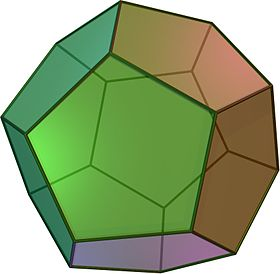# Resistors On A DodecahedronEach edge of a regular dodecahedron is a $1 \Omega$ resistor. If the effective resistance between two adjacent vertices can be represented as $\dfrac {a}{b} \Omega$ where $a$ and $b$ are co-prime positive integers, find $a + b$.

A dodecahedron has 20 vertices and 30 edges with 3 edges meeting at each vertex.

×